# Numbers and Constructibility

This article follows my article on the construction of numbers where I introduced natural, rational, real and complex numbers. It’s not necessary to have read it though. Here, I’ll present irrational, constructible, algebraic, transcendental, computable and definable numbers.

Last summer, I visited the exposition Dynamo at the Grand Palais in Paris. Some of the beautiful art pieces were mind-blowing mathematical objects! I got particularly intrigued by one artwork by François Morellet…

What was it?

It’s very simple. It consists in superposing 4 grids inclined by angles of 0, 22.5°, 45° and 67.5°. This is what you obtain:I stood 10 long minutes in front of this painting. Speechless, and deeply puzzled.

Really? What do you see in this painting? I don’t see anything…

Precisely! Given how simple its construction is, I was expecting strong patterns appearing. Yet, all we can see are chaotic unstructured messy lines!

Yes, you’re right! That is puzzling…

And, then, suddenly, it hit me.

What? What hit you?

What I was looking at, and what you are looking at, is the geometry of irrational numbers, including and especially the geometry of the square root of 2! In this article, I’ll show you what this irrationality means and why it generates such a chaotic picture. This will also give a Historical key argument to the existence of irrational numbers. After discovering these irrational numbers, mathematics has been building up more and more sophisticated classes of numbers I’ll then get to.

## Irrational Numbers

As you’ve probably learned it a long time ago, many numbers can be written as fractions. For instance, $2/3$, $7/5$, $241531/2353$, but also $3=3/1$. These are all fractions. In number theory, we call them rational numbers, as they can be expressed as a ratio. By opposition, irrational numbers are…

numbers which can’t be written as ratios!

Exactly!

But do these numbers even exist?

Back in Ancient Greece, the Pythagorean philosophers believed that all numbers were rationals. They could not stand the idea that there were numbers which couldn’t be written as fractions. But, Hippasus of Metapontum discovered that $\sqrt{2}$, the square root of 2, was in fact not rational. As the story goes, the Pythagoreans drowned him for this act of treason against his own community! This is what Marcus du Sautoy explains in this extract from the wonderful BBC show The Story of Maths:

So why can’t $\sqrt{2}$ be written as a fraction?

Humm… let’s get to that later…

OK… So what does the irrationality of $\sqrt{2}$ have to do with Morellet’s painting?

Let’s take a simpler version of Morellet’s painting. Namely, let’s remove the grids rotated by 22.5° and 67.5°. If we only keep the initial grid and the one inclined by 45°, here’s what we get: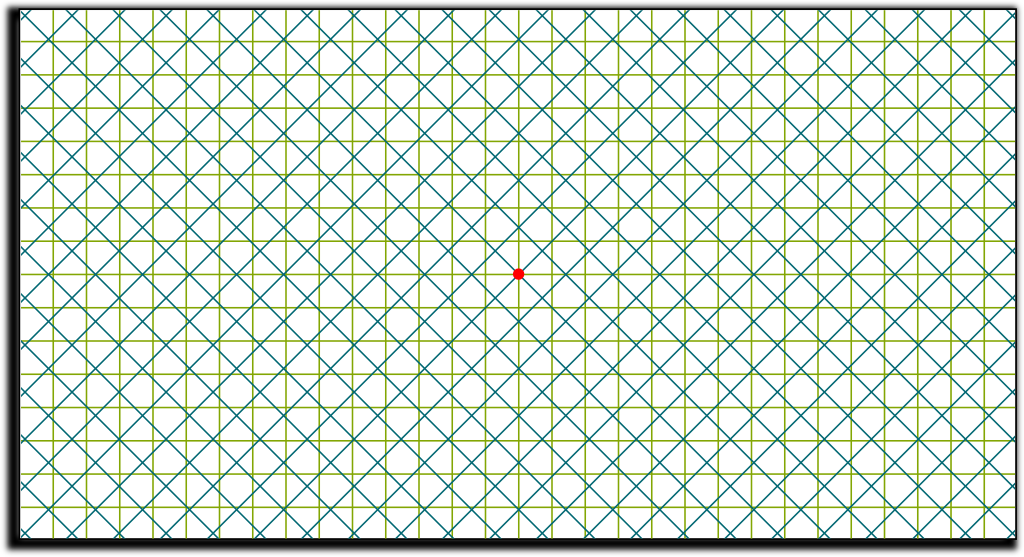Once again, except for the axial symmetries along the lines passing through red point where the two grids meet, there is no pattern appearing. In fact, as I’m staring at this patternless image longly, I’m getting dizzy…

Still, this doesn’t tell me what $\sqrt{2}$ has to do with this painting…

Amazingly, there is so little pattern that I can assure you that the two grids will never ever meet again. In fact, if you look at a large number of intersections relatively to each of the green squares, they will seem randomly and uniformly distributed! And the cause of that is precisely the irrationality of $\sqrt{2}$!

Why?

Let’s consider that all squares of the grids have a side of length 1 unit. To make matters simple, let’s only focus on the horizontal green line which goes through the red point, and let’s keep track of the intersections of the blue grid with this green line on the right of the red point. At the next intersection, the green line will have been the diagonal of a blue square. But as Marcus du Sautoy said, and as you can prove it using Pythagorus theorem, the diagonal of a square of side 1 is…

$\sqrt{2}$!

Exactly! And this means that the next intersection occurs $\sqrt{2}$ units away from the red point. But this pattern keeps repeating itself. The second intersection will occur $\sqrt{2}+\sqrt{2} = 2\sqrt{2}$ units away from the red point. Eventually, the $n$-th intersection occurs $n\sqrt{2}$ units away from the red point. But for the $n$-th intersection to be the intersection of the two grids, this intersection must occur at a whole number of units away from the red point. So, $n\sqrt{2}$ must be an integer $m$. But this can’t happen, as it would imply that $\sqrt{2}=m/n$, which would contradict…

the irrationality of $\sqrt{2}$! Waw!

I know! And you could go further and prove that the irrationality of $\sqrt{2}$ implies that the relative position of the intersections of the blue grid with the horizontal line with regards to green squares is dense.

By that I mean that the fractional part of $n\sqrt{2}$ is dense in $[0,1]$.

In fact, in the whole picture, if it is large enough, it means that if you pick randomly an point of the blue grid, then it will be uniformly distributed in the area of the green square which contains it! These facts describe mathematically how profoundly messy and chaotic Morellet’s brilliant artwork is! And this is why I assert that it displays the irrationality of $\sqrt{2}$…

The other grids of Morellet’s artwork are fundamentally related to other numbers, namely $\sin(\tau/16)$ and $\cos(\tau/16)$, where $\tau = 2\pi$ represents a full turn. Yes, just like Vihart, I prefer $\tau$ over $\pi$.
Can you tell me now why $\sqrt{2}$ is irrational?

Arg… I thought (hoped?) you had forgotten about that! Well, I’m feeling a bit lazy, so I’ll let the professors of Numberphile explain that, along with some other pretty cool facts about $\sqrt{2}$:

Wait! I’m at work, and I can’t watch the video because my boss is allergic to maths!

Well, he’s a bad boss! But, fine… Below is the proof. But don’t worry if you don’t understand it, it won’t be relevant for this article!

By contradiction, if $\sqrt{2}$ was rational, it could be written as an irreducible fraction $p/q$. We would then have $2 = \sqrt{2}^2 = p^2/q^2$, and thus $2q^2=p^2$. So, $p^2$ is even, which proves that $p$ is. Hence, $p^2$ is divisible by $4$. But then, $2q^2$ is divisible by $4$, thus $q^2$ by $2$ and $q$ is even too. So $p$ and $q$ are both even… which can’t happen as $p/q$ is irreducible! So our premise is wrong: $\sqrt{2}$ is irrational. By the way, this proof works also for any square root which is not integer!

## Constructible Numbers

While the Pythagoreans argued that irrational numbers don’t exist, Hippasus would argue that $\sqrt{2}$ does, because we can draw it. Nowadays, this argument doesn’t seem relevant as we have got used to dealing with so many more irrational numbers. Yet, it’s a crucial one in the History of mathematics. In particular, the expression squaring the circle comes from the fascinating concept of constructible numbers.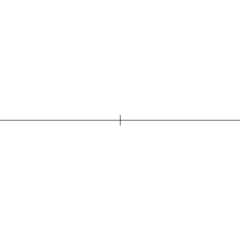What’s a constructible number?

It’s a number that can be constructed by using only a compass and straightedges.

What do you mean?

We start with two points in the plane. Then, to construct new points we must follow the three following rules:

1. Any new point we construct needs to be the intersection of two lines, two circles or a line and a circle.
2. Lines are drawn with the ruler and must pass through two points we have already constructed.
3. Circles must be centered on points we have already constructed, and their radius must be the distance between two points we have already constructed.
What does this have to do with numbers?

We call 1 the distance between the two initial points we had. Then, a number is constructible if we can construct two points such that the distance separating them is the number in question.

Can you give an example?

Sure! Let’s start by constructing all integer numbers. Call $A$ and $B$ the two initial points in the plane. Now, let’s draw this line with the ruler. Then, using the compass, we draw circles of radii 1, centered on $A$ and $B$. The new intersections we then obtain are points $A_1$ and $B_1$. Both are on the line $(AB)$. One is at distance 1 of $A$, and the other at distance 1 of $B$. By now repeating this procedure with circles of radii 1 centered on $A_1$ and $B_1$ and so on, we can in fact construct all points on $(AB)$ at an integer distance from $A$ and $B$. This is what’s done below: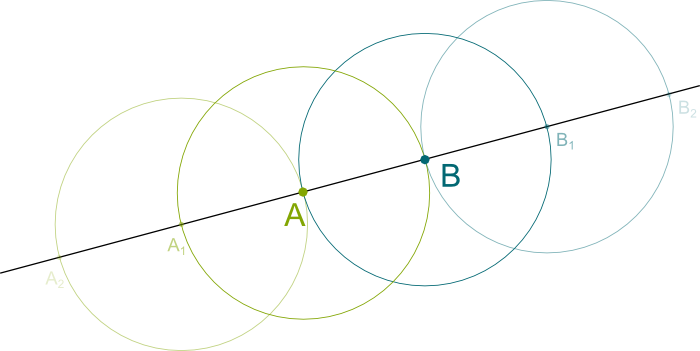So integer numbers are constructible… What about $\sqrt{2}$?

Hehe… This is getting interesting! Let’s first show you how to construct a perpendicular to a line that goes through a certain point. This will be helpful to construct $\sqrt{2}$!

So how do you construct a perpendicular?

Consider a line we have already constructed. This means that there are two points $A$ and $B$ of this line we have constructed. Let $C$ the point we want the perpendicular to go through. Then the circle of radius $AC$ and center $A$, and the circle of radius $BC$ and center $B$ meet in $C$ as well as in another point $D$. The line that goes through $C$ and $D$ is then the perpendicular we were searching for!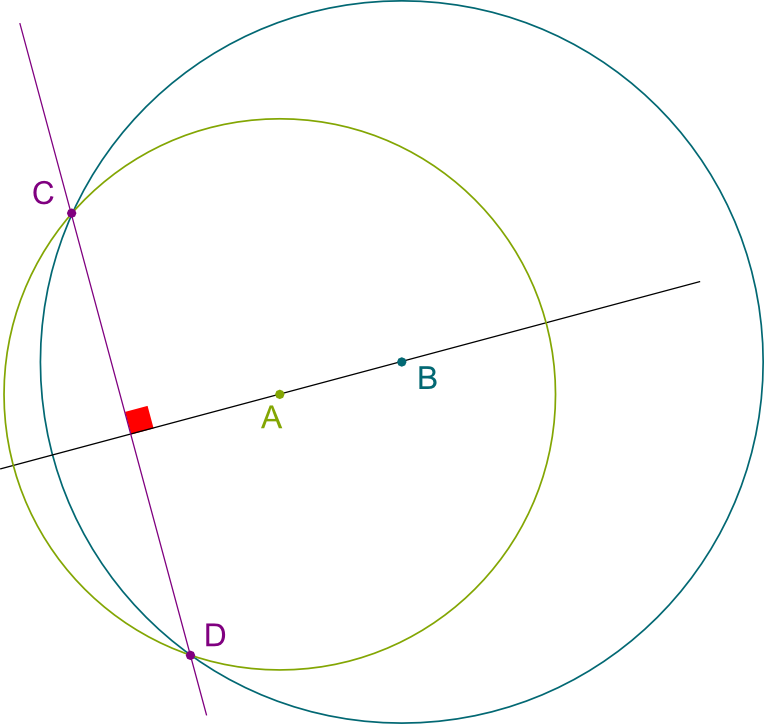To prove that the line $(CD)$ is indeed a perpendicular, one way consists in showing that the figure above is symmetric with regards to the line $(AB)$. However, this construction fails if $C$ is lying on the line that goes through $A$ and $B$. I’ll leave it to your ingenuity to figure out how to do the construction of the perpendicular in that case!

Combining the constructions of integer numbers and of perpendiculars that go through a certain point, we can now construct a grid like the one this article started with, whose squares are of side 1! In particular, any square of this grid has a diagonal of length $\sqrt{2}$… We have just proved that $\sqrt{2}$ is constructible!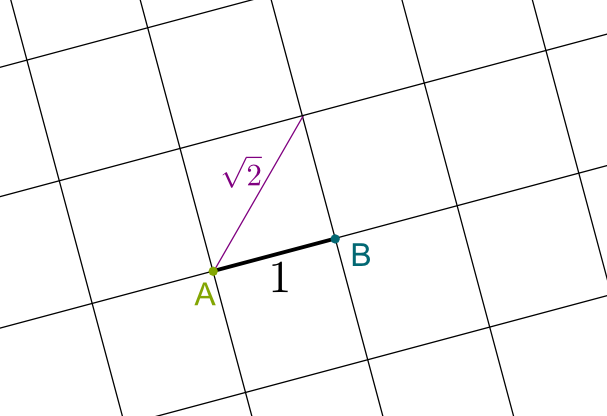What about rational numbers? Are they constructible?

Yes there are! Let’s consider $3/5$ for instance. In the grid, we can consider a rectangle of width 5 and height 3. Once again, we will draw its diagonal. This diagonal intersects the first of the 5 vertical lines at height $3/5$! And if you want any rational number $p/q$, you simply need to do this for a rectangle $p$ by $q$ instead!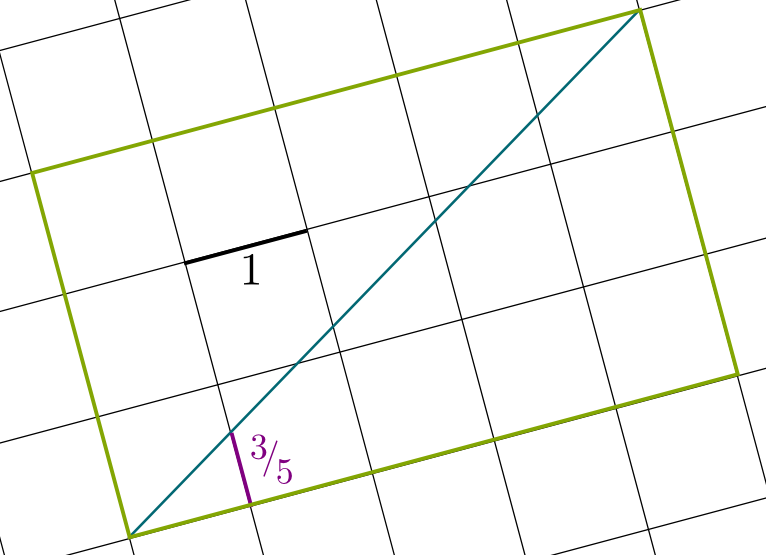The proof that we indeed obtain $p/q$ is a direct consequence of the intercept theorem.
What about square roots other than $\sqrt{2}$? Are they all constructible too?

Yes! For instance, to construct $\sqrt{5}$, consider a circle of diameter $5+1=6$, centered on a point of the grid. Then, the intersection of the vertical line at distance 1 from the intersection of the horizontal diameter with the circle defines a height of $\sqrt{5}$. And once again, to construct any $\sqrt{n}$, we just need to take a circle of diameter $n+1$! This is what’s done below: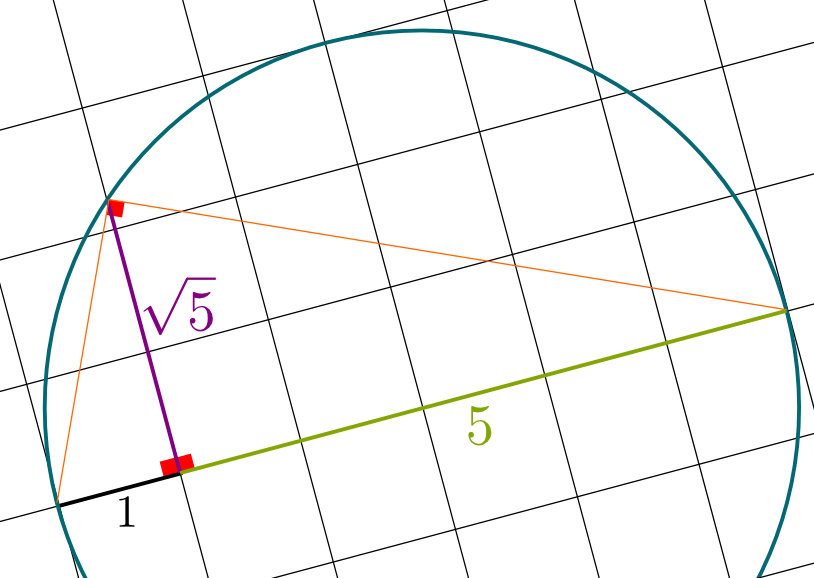To prove that we have indeed constructed $\sqrt{n}$, you need to apply the Pythagorean theorem to the three rectangle triangles whose right angles are depicted on the picture!

In fact, this construction shows that we can construct the square root of any constructible numbers. So, not only is $\sqrt{5}$ constructible, but so is $\sqrt{5} = \sqrt{\sqrt{5}}$, as well as other more complicated numbers such as $\sqrt{\sqrt{2}+\sqrt{3}}$!

Waw! Is there even some number that’s not constructible?

That’s a great question! Historically, the regular heptagon, the regular polygon of 7 sides, was the first polygon to be shown to be non-constructible!

Really? You’re saying that a regular heptagon can’t be constructed with a compass and a ruler? That’s surprising!

I know! This is known as the Gauss-Wantzel theorem. And it implies that the numbers associated to the heights of the points of a regular heptagon of side 1 are non-constructible! More generally, the beautiful Galois theory has emerged around the 1830s to give an amazing insight into the constructibility, algebra and geometry of numbers! Using it, it’s relatively simple to then show that $\sqrt{2}$ is non-constructible! Unfortunately, I won’t have time to get into it here… But I’ll definitely write about it soon!

What about the most important number of mathematics, $\pi$. Is it constructible?

It took a while to figure it out, but the most important number of mathematics, $\tau$ (and not $\pi$!), is non-constructible! This non-constructibility corresponds to the impossibility of squaring the circle, as explains by the awesome James Grime on Numberphile:

## Transcendental Numbers

Most greek scholars considered that numbers needed to be constructible to exist. But the invention of algebra by Muslim scholars then showed that numbers can be defined in other ways than with a compass and a straightedge! In particular, that’s the case of $\sqrt{2}$. This led mathematician to define a new class of numbers, called the algebraic numbers.

What’s an algebraic number?

An algebraic number is a number constructible by a finite number of algebraic manipulations. More precisely, it’s a number which can be brought to 0 with a finite number of multiplications and additions. This is what’s brilliantly explained by Simon Pampena on Numberphile:

So, as explained by Simon, another way of defining algebraic numbers is by considering them as the solutions of polynomial equations. These are equations of the form $a_n x^n + a_{n-1} x^{n-1} + \ldots + a_1 x + a_0 = 0$, where the coefficients $a_i$ are integers. For instance, when $n=3$, $a_3=1$, $a_2 = a_1 =0$ and $a_0=-2$, the polynomial equation becomes $x^3-2=0$. Since $\sqrt{2}$ is a solution, it is an algebraic number.

It’s not the only solution though, is it?

Amusingly, no. This equation has other solutions, namely $\sqrt{2} e^{i\tau/3}$ and $\sqrt{2} e^{2i\tau/3}$.

If you’re not familiar with complex numbers, check my article on the topic!

Galois theory defines these other solutions as the conjugates of $\sqrt{2}$. This means that, whenever we replace $\sqrt{2}$ by any of the two others in algebraic equalities, the equalities still hold. Thus, from a purely algebraic viewpoint, that is, if we only consider additions, multiplications and equalities, there is no difference between $\sqrt{2}$ and its conjugates.

More precisely, for any algebraic number, there is a polynomial equation of smallest degree it is the solution of. The conjugates are the other solutions to that polynomial equation. Galois theory then focuses on the symmetries between the conjugates. In particular, understanding the group $Gal(\overline{\mathbb Q}/\mathbb Q)$ of all symmetries between algebraic numbers is a very active field of research! And the success of the theory is already symbolized by Andrew Wiles’ use of group representation on $Gal(\overline{\mathbb Q}/\mathbb Q)$ to prove Fermat’s last theorem.
So, I guess that algebraic numbers are numbers which can be written as combinations of $n$-th roots, right?

Actually, no. A famous surprising result of Galois theory is that most algebraic numbers can actually not be written with radicals. In particular, for equations of degree at least 5, there is no way to write the solutions as a combination of $n$-th roots of the coefficients. And this is the case of the only real number solution to the equation $x^5-x-1=0$, which is about $1.1673$, and is displayed in the following figure: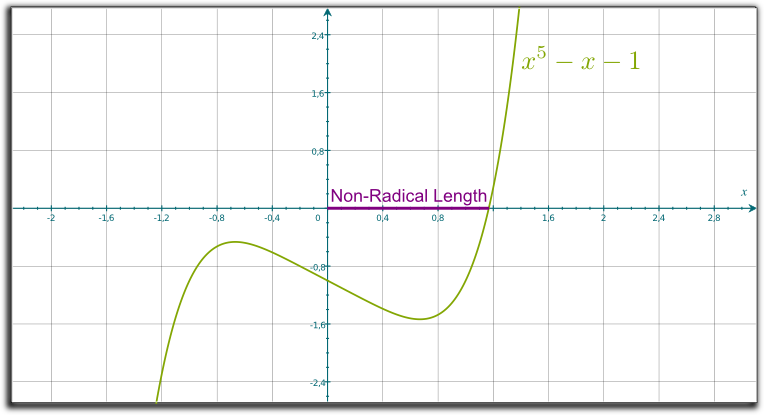So, as James and Simon both said it, $\pi$ is not algebraic?

Indeed, it is not. Nor is $\tau = 2\pi$. For this reason, it is called a transcendental numbers. The other important example is Euler’s constant $e \approx 2.7$. Numbers constructed from $\pi$ and $e$ are often transcendental too. In particular, Hilbert’s 7th problem has been solved in 1934 by the Gelfond-Schneider theorem. It states that if $a$ is algebraic different from 0 and 1 and if $b$ is irrational algebraic, then $a^b$ is transcendental.

Technically, $a^b$ is defined as $e^{b \ln a}$. Since the complex logarithm is actually defined up to addition by $i\tau$, $a^b$ is actually multivalued. The Gelfond-Schneider then says that all the values of $a^b$ are transcendental.

## Computable Numbers

Crucially, transcendental numbers are not constructible geometrically nor algebraically…

Wait… So how are these transcendental numbers even defined?

Hehe… Great question! Most are in fact defined analytically. For instance, $\tau$ is typically defined as one of the solutions of the non-polynomial equation $\sin(\tau)=0$. More simply, transcendental numbers are often defined as infinite series like $e = \sum 1/n! = 1+1/1+1/2+1/6+1/24+\ldots$. In fact, the invention of infinite sums has led to a boom of constructed numbers in mathematics.

Like what?

Historically, the first number to be proved transcendental is the Liouville constant $\sum 10^{-n!}$. Another more recent example is the Champernowne constant $0.123456789101112…$, which can be precisely written using an infinite sum.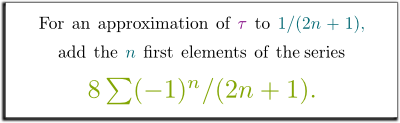So, is there a name for numbers which are constructible analytically?

Sort of. In 1936, British mathematician Alan Turing defined computable numbers as numbers which can be approximated as closely as desired by a finite terminating procedure. Infinite series $\sum a_n$, whose speed of convergence we can control and whose general terms $a_n$ are all given by the same finite terminating procedure, are computable.

More precisely, a number is computable if there exists a Turing machine which, when fed with a number $n$, returns in finite time the $n$-th digit of the number. Equivalently, it is computable if there exists a Turing machine which, when fed with a rational number $q$, returns in finite time an approximation of the number at distance less than $q$.
Are there examples of real numbers which are not computable?

Yes! Historically, the first non-computable real number envisioned was introduced by French mathematician Émile Borel in 1927. Around the same time as Kurt Gödel, Borel noticed that there is only a countably infinite amount of yes-no questions we can ask with words or mathematical symbols. Thus, Borel imagined listing them all, and devised a single real number to include all the answers of all yes-no mathematical questions: The $n$-th decimal digit of this number in base 2 would simply be 0 if the answer of the $n$-th question is no, and it would be 1 otherwise.

So Borel’s number would contain all the mathematics? Waw! Can we do that?

Yes! Isn’t it amazing? Unfortunately though, this number is still hard to define as we need to come up with an actual way of listing all the mathematical questions. But, building up on this idea, Gregory Chaitin defined uniquely and unambiguously a similar kind of number, now known as Chaitin’s constant, and commonly denoted $\Omega$.

What’s that number?

Roughly speaking, $\Omega$ is real number between 0 and 1 which represents efficiently all the information about the termination of an algorithm for some randomly chosen input. It thus depends on the algorithm we consider. But for almost all algorithms, this number is non-computable.

More precisely, the algorithm I’m referring to must be a universal Turing machine you can read about in my article on the P vs NP problem. Each input $p$ of length $|p|$ is given a weight $2^{-|p|}$. By considering that there is a sequence of characters which end inputs, we make sure that $\sum 2^{-|p|}$ is less than 1. Chaitin’s constant is then defined as the sum of $2^{-|p|}$ when the inputs $p$ correspond to a terminating algorithm.
So why is $\Omega$ non-computable?

To have an approximation of $\Omega$, we need to test whether inputs of small lengths have the algorithm terminating. Yet, this corresponds to solving many halting problems, which have been proved to be non-computable!

Is $\Omega$ algebraic?

No. And that’s because all algebraic are computable!

$\Omega$ is a truly mind-blowing number which deserves its own article. For instance, Chaitin proved that, even in theory, using today’s mathematical framework can only determine a finite number of its decimals! In fact, by considering the right universal Turing machine, you can make sure that all digits of $\Omega$ are not computable! If you can, please write about it!
So, is there a class of numbers $\Omega$ is in?

Yes! $\Omega$ is a definable number, which means that it can be defined with a finite number of words or symbols in a mathematical theory. Thus, definable numbers contain by definition exactly all the numbers we will ever be able to construct.

Here is where I should confess the limits of my knowledge, and, visibly, wikipedia has been misleading me! What I’ve understood after a talk with Andrew Cave is that a mathematical theory is actually a framework which talks about many possible cases. So, kind of like when I talk about even numbers I am addressing them all, our whole usual mathematical theory, called ZFC, talks about many kinds of “actual” mathematics. These actual mathematics are called models. Weirdly enough, it has been proven that, if ZFC is consistent, there exists a model in which objects are simply their mathematical definitions (or proofs of existence and uniqueness). Interestingly, you can think of this model as bag of apples, where apples stand for objects. Weirdly enough, in this bag, there are countably many apples… And yet, it does include all real numbers! And the explanation of that lies in the misconception that countability has to do with size. In its heart, it rather is about the existence of bijective map, which must be an apple of the bag. So, the reason why real numbers are still uncountable is because there are missing apples in the bag to connect them with natural numbers. But, from outside the model, we can see that the whole bag only has a countable number of objects. And thus of real numbers… So, within the model, real numbers are uncountable, but they are from an outside perspective. Now, in this setting, all real numbers are in fact definable… Troubling, right?

## Let’s Conclude

To recapitulate all we’ve talked about, here’s a Venn diagram which depicts all the classes of numbers we have discussed in this article: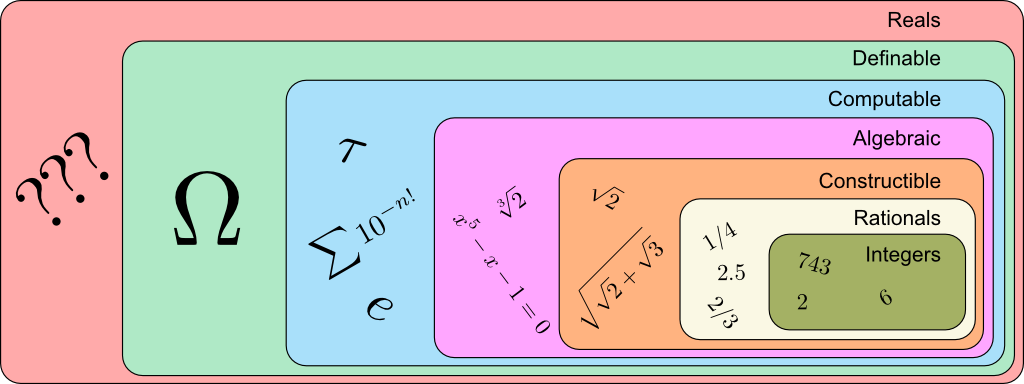As you can see, the realm of numbers mathematics can define has kept increasing, using sequentially geometric, algebraic, analytic and set theoretical arguments! Amusingly though, the set mathematicians are the most familiar is none of these. Indeed, the numbers mathematicians most often refer to is the set of real numbers, which, for the overwhelming majority, are actually fundamentally non-constructible…

Finally, I’ll conclude with a few words about what it means to be a mathematician. Last summer, I visited the Grand Palais with my mom. She’s awesome (obviously!), but she didn’t get as puzzled as I did when facing Morellet’s artwork. And this made me realize what the most important quality of a mathematician was: It’s the ability to be deeply intrigued, almost obsessed, by patterns. In some sense, this is the best definition I know of what a mathematician is.

## More on Science4All

The Magic of AlgebraBy Lê Nguyên Hoang | Updated:2016-02 | Views: 3817
The power of algebra lies in abstraction, and abstraction is basically forgetting. By retracing the History of algebra from its roots to more recent advancements, this article unveils the numerous breakthrough in our understanding of the world, by abusing of the power of forgetting.

Construction and Definition of NumbersBy Lê Nguyên Hoang | Updated:2016-02 | Views: 9073
Although they have been used for thousands of years, an actual definition of numbers was given less than a century ago! From the most fundamental level of set theory, this article takes you to the journey of the construction of natural, integer, rational, real and complex numbers.

Imaginary and Complex NumbersBy Lê Nguyên Hoang | Updated:2016-02 | Views: 6799
My first reaction to imaginary numbers was... What the hell is that? Even now, I have trouble getting my head around these mathematical objects. Fortunately, I have a secret weapon: Geometry! This article proposes constructing complex numbers with a very geometrical and intuitive approach, which is probably very different from what you've learned (or will learn).

The Most Beautiful Equation of Math: Euler's IdentityBy Lê Nguyên Hoang | Updated:2016-01 | Views: 93205
In 1988, Euler's identity was elected most beautiful theorem of mathematics. It has been widely taught worldwide. But have you ever stopped to really sense the meaning of this incredible formula? This article does.

The Harmonious Mathematics of MusicBy Lê Nguyên Hoang | Updated:2015-12 | Views: 16302
It was when hearing the sounds of hammers that Pythagoras realized the ubiquity of numbers in mathematical harmony. He would go on laying down the mathematical foundations of music, based on octaves, perfect fifths and major thirds. This mathematics of music would then become the favourite playground of all musicians, from Beethoven to Gangnam Style.